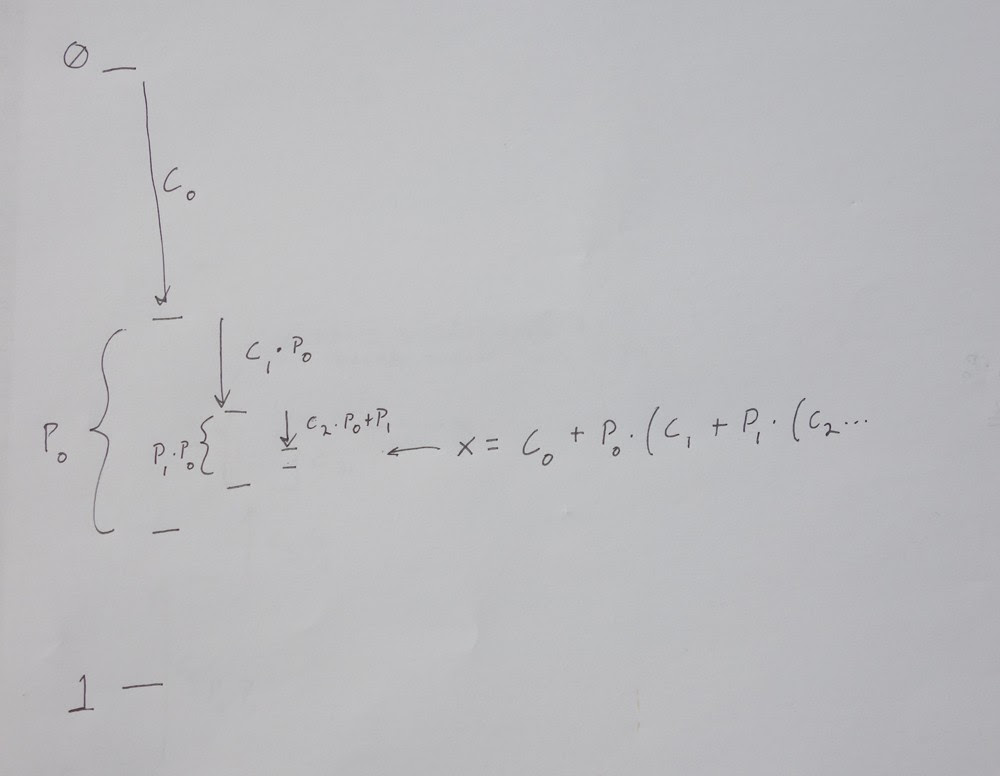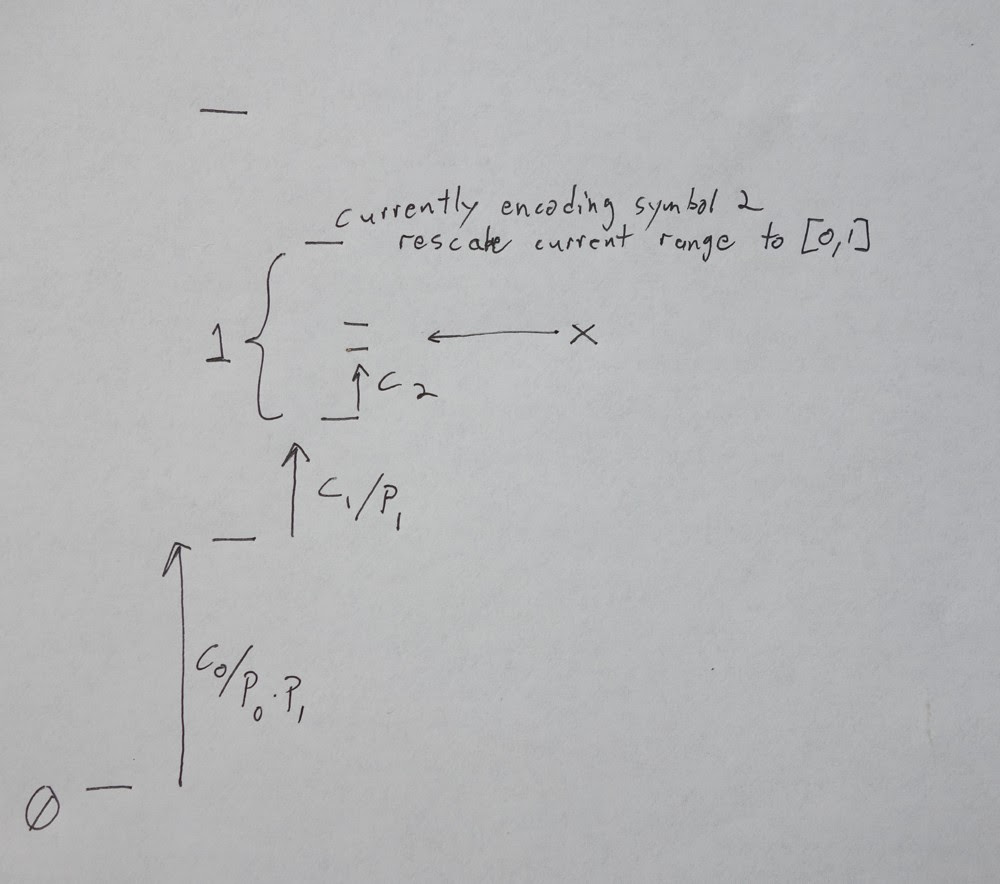# cbloom rants

## 2/02/2014

### 02-02-14 - Understanding ANS - 4

Another detour from the slow exposition to mention something that's on my mind.

Everyone is familiar with the standard simplified idea of arithmetic coding. Each symbol has a probability P(s). The sum of all preceding probability is the cumulative probability, C(s).

You start with an interval in [0,1]. Each symbol is specified by a range equal to P(s) located at C(s). You reduce your interval to the range of the current symbol, then put the next symbol within that range, etc. Like this :As you go, you are making a large number < 1, with more and more bits of precision being added at the bottom. In the end you have to send enough bits so that the stream you wanted is specified. You get compression because more likely streams have larger intervals, and thus can be specified with fewer bits.

In the end we just made a single number that we had to send :

```
x = C0 + P0 * ( C1 + P1 * (C2 ...

```
in order to make that value in a FIFO stream, we would have to use the normal arithmetic coding style of tracking a current low and range :
```
currenty at [low,range]

low <- low + range * Cn
range <- range * Pn

```
and of course for the moment we're assuming we get to use infinite precision numbers.

But you can make the same final value x another way. Start at the end of the stream and work backwards, LIFO :

```
LIFO :

x contains all following symbols already encoded
x in [0,1]

x' <- Cn + Pn * x

```
there's no need to track two variables [low,range], you work from back to front and then send x to specify the whole stream. (This in fact is an ancient arithmetic coder. I think it was originally described by Elias even before the Pasco work. I mention this to emphasize that single variable LIFO coding is nothing new, though the details of ANS are in fact quite new. Like "range coding" vs prior arithmetic coders , it can be the tiny details that make all the difference.) (umm, holy crap, I just noticed the ps. at the bottom of that post ("ps. the other new thing is the Jarek Duda lattice coder thing which I have yet to understand")).

This is the clearest one I've found : ibmrd2302G.pdf "Arithmetic Coding" , Rissanen & Langdon, IBM J Res. D, March 1979 The "dual" Elias code uses a single state variable and is the same as the LIFO arithmetic coder I describe. The non-dual is the forward two-variable version. In equation 4, P is a cumulative probability (my C), and l (lower case) is the length of a codeword. Let l(k)=-log2(p(k)) then C_bar <- P(k) + p(k) * C_bar is the single-variable LIFO Elias coder.

You can decode an individual step thusly :

```
x in [0,1]
find s such that C(s) <= x < C(s+1)

x' <- (x - Cs)/Ps

```

Now let's start thinking about doing this in finite precision, or at least fixed point.

If we think of our original arithmetic coding image, growing "x" up from 0, instead of keeping x in [0,1] the whole time, let's keep the active interval in [0,1]. That is, as we go we rescale so that the bottom range is [0,1] :That is, instead of keeping the decimal at the far left and making a fraction, we keep the decimal at the far right of x and we grow the number upwards. Each coding step is :

```
x' <- x/Ps + Cs

```
in the end we get a large number that we have to send using log2(x) bits. We get compression because highly probable symbols will make x grow less than improbable symbols, so more compressable streams will make smaller values of x.

(x = the value before the current step, x' = the value after the current step)

We can decode each step simply if the (x/Ps) are integers, then the Cs is the fractional part, so we just do :

```
f = frac(x)
find s such that C(s) <= f < C(s+1)

x' <- (x - Cs)*Ps
```
that is, we think of our big number x as having a decimal point with the fractional part Cs on the right, and the rest of the stream is in a big integer on the left. That is :
```
[ (x/Ps) ] . [ Cs ]

```

Of course (x/Ps) is not necessarily an integer, and we don't get to do infinite precision math, so let's fix that.

Let :

```
Ps = Fs / M

P is in [0,1] , a symbol probability
F is an integer frequency
M is the frequency denominator

Sum{F} = M

Cs = Bs / M

B is the cumulative frequency

```
now we're going to keep x an integer, and our "decimal" that separates the current symbol from the rest of the stream is a fixed point in the integer. (eg. if M = 2^12 then we have a 12 bit fixed point x).

Our coding step is now :

```
x' = x*M/Fs + Bs

and we can imagine this as a fixed point :

[ (x*M/Fs) ] . [ Bs ]

in particular the bottom M-ary fraction specifies the current symbol :

( x' mod M ) = Bs

```
the crucial thing for decoding is that the first part, the (x/P) part which is now (x*M/F) shouldn't mess up the bottom M-ary fraction.

But now that we have it written like this, it should be obvious how to do that, if we just write :

```
x*M/F -> floor(x/F)*M

then the (mod M) operator on that gives you 0, because it has an explicit *M

```
so we've made the right (x/P) scaling, and made something that doesn't mess up our bottom mod M for decodability.

But we've lost some crucial bits from x, which contains the rest of the stream. When we did floor(x/F) we threw out some bottom bits of x that we can't get rid of. So we need that (x mod F) back.

Fortunately we have the perfect place to put it. We can specify the current symbol not just with Bs, but with anything in the interval [Bs , Bs + Fs) ! So we can do :

```
x' = M*floor(x/Fs) + Bs + (x mod Fs)

which is :

x' = [ floor(x/Fs) ] . [ Bs + (x mod Fs) ]

with the integer part growing on the left and the base-M fractional part on the right specifying the current symbol s

```
and this is exactly the rANS encoding step !

As we encode x grows by (1/P) with each step. We wind up sending x with log2(x) bits, which means the code length of the stream is log2(1/P0*P1...) which is what we want.

For completeness, decoding is straightforwardly undoing the encode step :

```
f = M-ary fraction of x  (x mod M)
find s such that Bs <= f < Bs+1

x' = Fs * (x/M) + (x mod M) - Bs

or

x' = Fs * intM(x) + fracM(x) - Bs

and we know

(fracM(x) - Bs) is in [0, Fs)

```
which is the same as the old arithmetic decode step : x' = (x - Cs)/Ps

Of course we still have to deal with the issue of keeping x in fixed width integers and streaming, which we'll come back to.